## Algebra

Showing posts with label restrictions. Show all posts
Showing posts with label restrictions. Show all posts

### Solving Rational Equations

Rational equations are simply equations with rational expressions in them.

Use the technique outlined earlier to clear the fractions of a rational equation.  After clearing the fractions, we will be left with either a linear or a quadratic equation that can be solved as usual.

Step 1: Factor the denominators.
Step 2: Identify the restrictions.
Step 3: Multiply both sides of the equation by the LCD.
Step 4: Solve as usual.
Step 5: Check answers against the set of restrictions.This process sometimes produces answers that do not solve the original equation (extraneous solutions), so it is extremely important to check your answers.
Tip: It suffices to check that the answers are not restrictions to the domain of the original equation.

Solve.Determining the LCD is often the most difficult part of the process. Use one of each factor found in all denominators.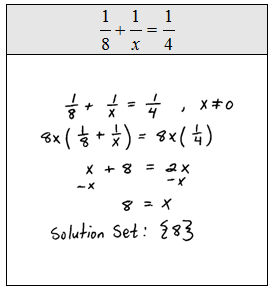Because of the distributive property, multiplying both sides of an equation by the LCD is equivalent to multiplying each term by that LCD as illustrated in the following examples.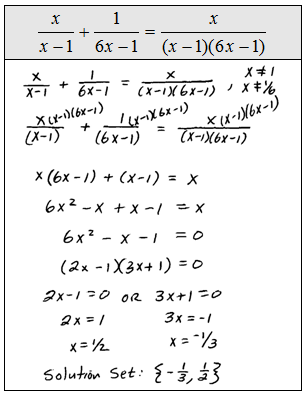Some literal equations, often referred to as formulas, are also rational equations. Use the techniques of this section and clear the fractions before solving for the particular variable.

Solve for the specified variable.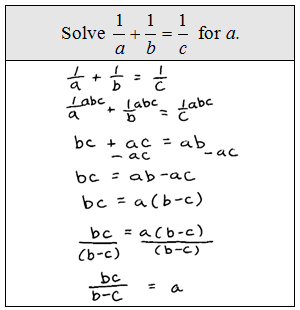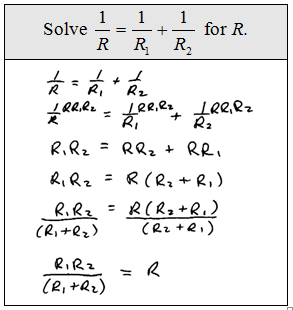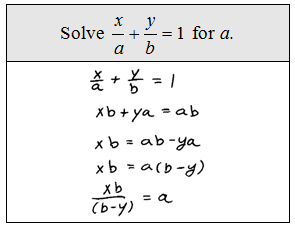The reciprocal of a number is the number we obtain by dividing 1 by that number.

Word Problem: The reciprocal of the larger of two consecutive positive odd integers is subtracted from twice the reciprocal of the smaller and the result is 9/35. Find the two integers.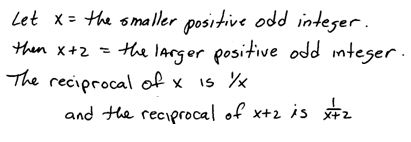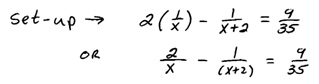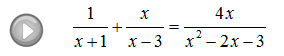### Simplifying Rational Expressions

Given a rational expression, the quotient of two polynomials, we will factor the numerator and denominator if we can and then cancel factors that are exactly the same.When evaluating rational expressions, plug in the appropriate values either before simplifying or after, the result will be the same.  Although, it is more efficient to simplify first then evaluate.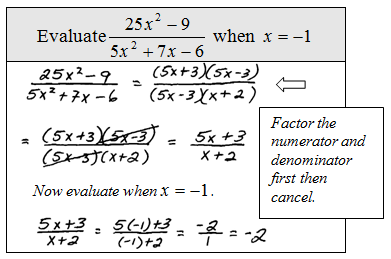We can see that when evaluating, the result will be the same whether or not we simplify first.  It turns out that not all numbers can be used when we evaluate.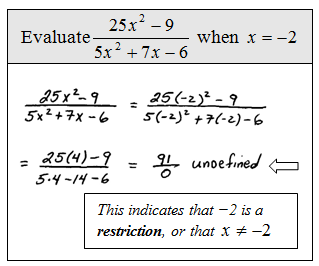The point is that not all real numbers will be defined in the above rational expression.  In fact, there are two restrictions to the domain, -2 and 3/5.  These values, when plugged in, will result in zero in the denominator.  Another way to say this is that the domain consists of all real numbers except for −2 and 3/5.

Tip: To find the restrictions, set each factor in the denominator equal to zero and solve. The factors in the numerator do not contribute to the list of restrictions.

Simplify and state the restrictions to the domain.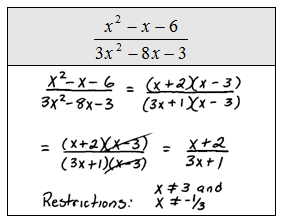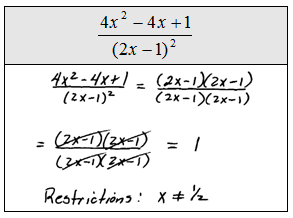Even if the factor cancels it still contributes to the list of restrictions.  Basically, it is important to remember the domain of the original expression when simplifying. Also, we must use caution when simplifying, please do not try to take obviously incorrect shortcuts like this: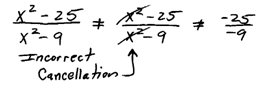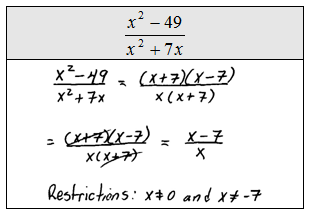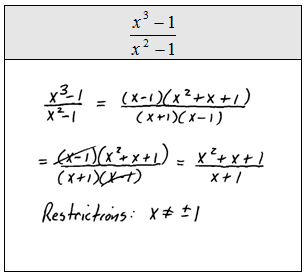Since subtraction is not commutative, we must be alert to opposite binomial factors.  For example, 5 − 3 = 2 and 3 − 5 = −2. In general,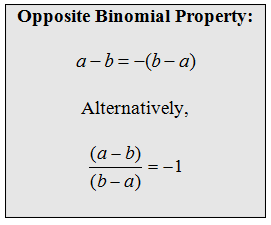Simplify and state the restrictions to the domain.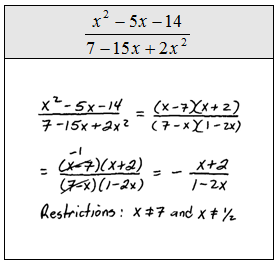At this point, we evaluate using function notation.### Elementary Algebra Exam #4

Click on the 10 question exam covering topics in chapter 7 (Rational Expressions and Equations). Give yourself one hour to try all of the problems and then come back and check your answers.

Simplify (Assume all denominators are nonzero.)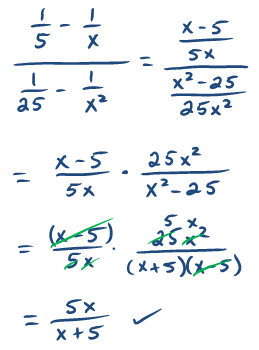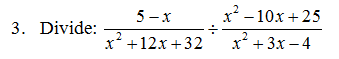Perform the operations and state the restrictions.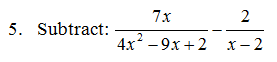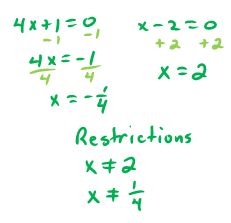Solve.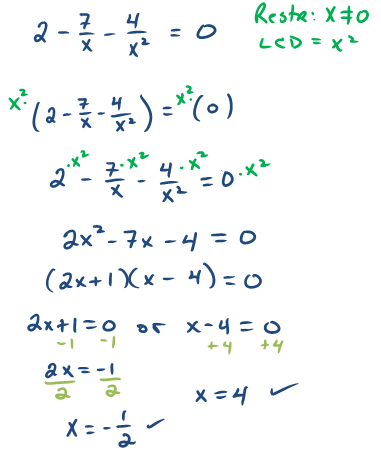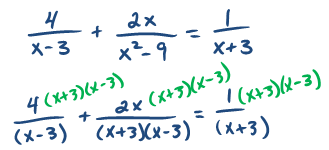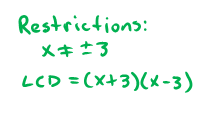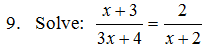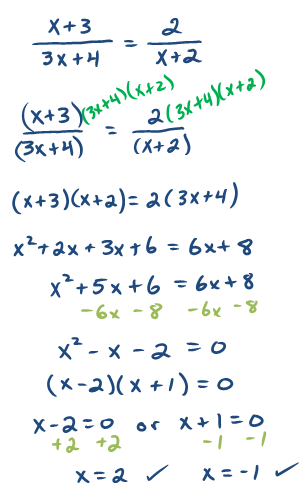10. The sum of the reciprocals of two consecutive odd integers is 4/3.  Set up an algebraic equation and use it to find the two integers.---